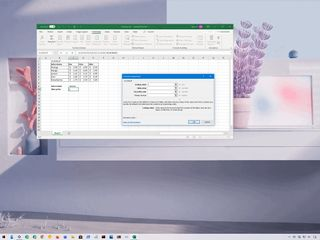# How to use VLOOKUP in Microsoft ExcelIn Microsoft Excel, VLOOKUP (vertical lookup) is a search function that you can use to find any data inside a particular column of the table by looking at the first column's entries and returning a corresponding value from another column.

While in a small table, you may be able to glance and quickly determine the information you need, it's different when working with an extensive spreadsheet with hundreds of rows and columns. Since you can spend a long time analyzing and finding the required information, Excel's VLOOKUP function was created to simplify data retrieval.

VLOOKUP works by performing a vertical search (top to bottom) for a value in the first column (that acts as the unique identifier), and then it returns a result from the matching row. The Excel function works like a drink menu at the coffee shop, where you start with the information you know, such as the drink's name, and then you look to the right to get the information you don't know, for example, the price.

In this Windows 10 guide, we'll walk you through the steps to correctly write a basic VLOOKUP function with the desktop version of Microsoft Excel, whether you use the version of Office available through a Microsoft 365 subscription, Office 2019, Office 2016, or earlier version.

## How to write VLOOKUP function in Excel

To write a VLOOKUP function manually in Excel, use these steps:

1. Open Excel.
2. Create the first column with items that will work as unique identifiers (required).
1. Create one or more additional columns (on the right side) with the different values for each item from the first column (on the left side).
1. Select an empty cell in the spreadsheet and specify the name of the item you want to find an answer to—for example, Orange.
1. Select an empty cell to store the formula and returned value.
2. In the empty cell, type the following syntax to create a VLOOKUP formula and press Enter:`=VLOOKUP()`
1. Type the following arguments inside the parenthesis "()" to write the function and press Enter:`=VLOOKUP(lookup_value,table_array,col_index_num,range_lookkup)`
• lookup_value: defines the cell that includes the product identifier from the first column on the left.
• table_array: defines the range of data where you want to perform a search. Typically, you would select the entire Excel table.
• col_index_num: defines the column number that the function will look to find a value. When specifying multiple columns, you should do from left to right.
• Quick note: If you don't specify a value, then the "true" option will be applied by default. Sometimes, when using the "true" option, the first column needs to be shorted, which may cause an unexpected result. If you're not getting the correct value, you should use the "false" option or sort the first column alphabetically or numerically.
In the command, make sure to update the variables inside the parenthesis with the information you want to query. Also, remember to use a comma to separate each value in the function. You do not need a space between each comma.Here's an example that returns the price for the 20oz bottle of orange juice:`=VLOOKUP(C10,B4:E8,4,FALSE)`

Once you complete the steps, the feature will return the value for the item you specified on step No. 4. If you receive the "#NAME?" error value, then it means that the formula is missing one or multiple quotes.

If you are trying to find data for another item, update the name of the cell on step No. 4. For example, if you want to see the price for the "20oz" bottle of Kiwi juice, then replace "Orange" with "Kiwi" in the "lookup_value" cell and press Enter to update the result.

## How to build VLOOKUP function in Excel

In addition to writing a formula directly into the spreadsheet, you can also use the Functions Arguments wizard, which gives you a more user-friendly interface to build the lookup formula.

To use the Function Arguments wizard to build a VLOOKUP formula in Microsoft Excel, use these steps:

1. Open Excel.
2. Create the first column with a list of items that will act as unique identifiers (required).
1. Create one or more additional columns (on the right side) with the different values for each item from the first column (on the left side).
1. Select an empty cell in the spreadsheet and specify the name of the item you want to find an answer to—for example, Orange.
1. Select an empty cell to store the formula and the returned value.
2. Click the Formulas tab.
3. Under the "Functions Library" section, click the Lookup and Reference drop-down menu and select the VLOOKUP option to open the Functions Arguments wizard.
1. In the Lookup_value field, specify the cell that contains the reference of the item you want to find the answer to—for example, C9.